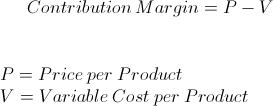# Contribution Margin

Contribution Margin Calculator (Click Here or Scroll Down)The formula for contribution margin is the sales price of a product minus its variable costs. In other words, calculating the contribution margin determines the sales amount left over after adjusting for the variable costs of selling additional products.

To better understand contribution margin, consider that the net income of a company is its revenues minus expenses. The term revenues is synonymous with sales, and expenses include fixed costs and variable costs. Fixed costs are expenses that typically do not change and are not heavily influenced by the quantity of products sold. Land and equipment are examples of fixed costs.

On the other hand, variable costs are more tied to the development of the product and is greatly affected by the number of products sold. Examples of variable costs are labor and materials.

The contribution margin helps to easily calculate the amount of revenues left over to cover fixed costs and earn profit.

## Example of Contribution Margin

Suppose that a company is analyzing its revenues and expenses. The company has sales of \$1,000,000 and variable costs of \$400,000. The contribution margin for this example would be the difference of \$1,000,000 and \$400,000, which is \$600,000. The \$600,000 is the amount left over to pay fixed costs. A 'per product' margin can be found by dividing \$600,000 by the number of units sold.

The actual calculation of contribution margin may be more laborious but the concept applies. See below for uses.

## Use of Contribution Margin Formula

Contribution margin is used by companies to simplify decisions regarding its operations. There are various ways that it could be applied. One application is using the contribution margin as a quick measure for break even analysis. The break even point for a company is when its revenues equal its expenses, leaving the company with neither a net profit nor net loss. For example, suppose that a company does a quick calculation for the contribution margin and finds that it is \$3 per product sold. If the company has \$30,000 in fixed costs for the period, then the break-even would be to sell 10,000 units for that same period. Contribution margin may also be used to compare individual product lines and also be estimated to set sales goals.

New to Finance?